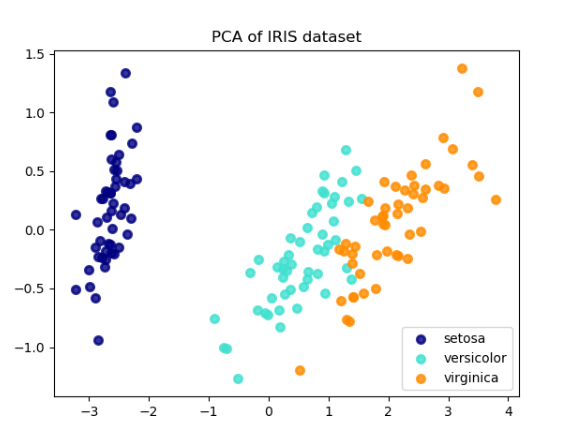top of page
Search

# Machine Learning Homework Help | Principle Component Analysis(PCA): Realcode4youMachine Learning Homework Help | principle component analysis

PCA is used to decompose a multivariate dataset in a set of successive orthogonal components that explain a maximum amount of the variance.

In other way we can say that:

It is used to reduce the dimensionality of such datasets, increasing interpretability but at the same time minimizing information loss.

For example reduce data from 2D to 1D and 3D to 2D, vice versa.

Below is an example of the iris dataset, which is comprised of 4 features, projected on the 2 dimensions that explain most variance:### Applications:

There are many applications of PCA which is given below:

• Facial recognition

• computer vision

• image compression

• And more other

It is also used in field of finance, data mining, bioinformatics, psychology, etc.

### Example 1: Using sklearn

# Principal Component Analysis

from numpy import array

from sklearn.decomposition import PCA

# define a matrix

A = array([[1, 2], [3, 4], [5, 6]])

print(A)

# create the PCA instance

pca = PCA(2)

# fit on data

pca.fit(A)

# access values and vectors

print(pca.components_)

print(pca.explained_variance_)

# transform data

B = pca.transform(A)

print(B)

### Output:

[[1 2]

[3 4]

[5 6]]

[[ 0.70710678 0.70710678]

[ 0.70710678 -0.70710678]]

[ 8.00000000e+00 2.25080839e-33]

[[ -2.82842712e+00 2.22044605e-16]

[ 0.00000000e+00 0.00000000e+00]

[ 2.82842712e+00 -2.22044605e-16]]

### Example 2: Without sklearn

Manually Calculate Principal Component Analysis.

Decompose 3*2 matrix into 3*1

#########code########

from numpy import array

from numpy import mean

from numpy import cov

from numpy.linalg import eig

# define a matrix

A = array([[1, 2], [3, 4], [5, 6]])

print(A)

# calculate the mean of each column

M = mean(A.T, axis=1)

print(M)

# center columns by subtracting column means

C = A - M

print(C)

# calculate covariance matrix of centered matrix

V = cov(C.T)

print(V)

# eigendecomposition of covariance matrix

values, vectors = eig(V)

print(vectors)

print(values)

# project data

P = vectors.T.dot(C.T)

print(P.T)

### Output:

[[1 2]

[3 4]

[5 6]]

[[ 0.70710678 -0.70710678]

[ 0.70710678 0.70710678]]

[ 8. 0.]

[[-2.82842712 0. ]

[ 0. 0. ]

[ 2.82842712 0. ]]

### Incremental PCA

The PCA object is very useful, but has certain limitations for large datasets. The biggest limitation is that PCA only supports batch processing, which means all of the data to be processed must fit in main memory.

Other way we can say, Incremental principal component analysis (IPCA) is typically used as a replacement for principal component analysis (PCA) when the dataset to be decomposed is too large to fit in memory.### Example:

#import Libraries

import numpy as np

import matplotlib.pyplot as plt

from sklearn.decomposition import PCA, IncrementalPCA

X = iris.data

y = iris.target

n_components = 2

ipca = IncrementalPCA(n_components=n_components, batch_size=10)

X_ipca = ipca.fit_transform(X)

pca = PCA(n_components=n_components)

X_pca = pca.fit_transform(X)

print(ipca.components_)

print(ipca.explained_variance_)

print(ipca.explained_variance_ratio_)

Output: